# Spatial Acoustics and Reverberation Time

## Visão geral

Spatial acoustic analysis is performed to determine the effect of the space or room on sound. Reverberation time measurement is one technique used to describe room acoustics by analyzing the decay of sound intensity. This tutorial details the measurements and analysis needed to find reverberation time.

## Reverberant Sound

Sound pressure waves are usually emitted from a source in all directions. In this picture are a few of the paths that these waves could have taken. Sound waves reflect off a surface and produce a resultant wave with less sound energy. This energy loss is due to the material composition of the surface that the sound wave was reflected from. Some energy of the wave is absorbed by the material, some is transmitted through the material while the remaining energy is reflected back into the space it originated from. This results in a decay of sound pressure in the space as the wave is reflected off many surfaces. This decay of sound pressure can become a very important factor in the acoustical quality of a space. If the purpose of a room was intended for speech or communication, a rapid decay of sound pressure would be needed to keep the speech intelligible. Imagine how difficult it would be to understand someone that was giving a speech in a space such as a pool in this figure.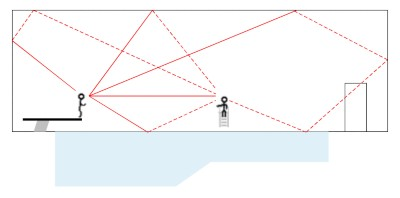Now, consider a large musical performance hall. In this space, a rapid decay of sound pressure would not be ideal, but rather a slow decay. This creates a very full sound that compliments the music, leaving tones that sound pleasing with the direct sound that the audience is focused on. The presence of reverberant sound gives musicians the ability to create very dynamic performances that are capable of filling the entire music hall and creating a dramatic experience for the audience.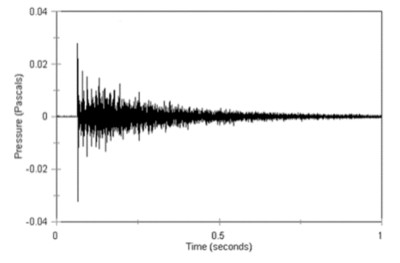## Reverberation Time

The length of time that it takes for the reverberant sound in a space to decay may need to be corrected depending on what the space is to be used for. A standard for measuring this time is referred to as Reverberation Time. Reverberation Time is defined as the amount of time required for the sound pressure in a space to decay 60 decibels, or to one millionth of the original energy level and can be approximated by the equation *T=0.049V/A T is the reverberation time in seconds and to put this time in perspective, reverberation time may range from around .6 seconds for a classroom to 1.5 -2 seconds for a concert hall. V is the volume of the space in cubic feet while A is the equivalent absorption area in square feet. As you can see from the equation, in a room with no absorption, T would go to infinity however, in practice all surfaces absorb some amount of sound energy which creates a finite reverberation time. In the chart, you can see the measured sound pressure decay of an impulse that occurred at time to. The time T that it took this disturbance to decay by 60 dB is then the reverberation time of the space.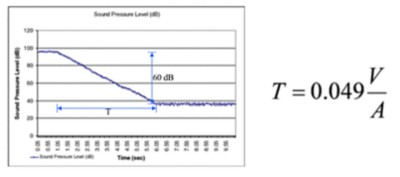## Equivalent Absorption Area

Imagine a space such as this cube constructed of different materials, each one absorbing a different amount of sound energy on each side of the cube. Infinite reflection is defined as a surface that would reflect all sound waves while infinite absorption is defined as a surface in which all sound would be absorbed. If for instance, if there were a sound source placed inside this cube, the area of an opening cut into the cube that would allow sound pressure to escape would be of infinite absorption. One can think of an the equivalent absorption area of the cube as the area of infinite absorption that could replace the area of the cube composed of materials with finite amounts of absorption. The equivalent absorption of each side of the cube is given as A=Sa, where “a” is the absorption coefficient of the material and “S” is the area in ft^2 of the material. Some common absorption coefficients will be listed later. The total equivalent absorption of the cube can be found by summing the equivalent absorption of all the surfaces of the space. If we sum the equivalent absorption of all the surfaces of the cube we can see that the area of the cube above is equivalent to 2 square feet of opening.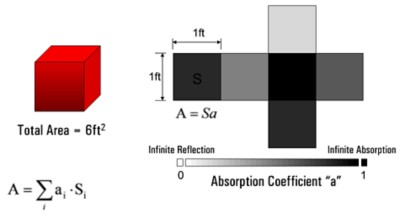One ft^2 of infinite absorption is defined as an absorption unit. Therefore the cube above contains 2 units of absorption.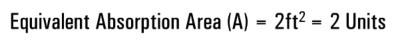## Common Absorption Coefficients

This table presents the value of the absorption coefficients for some common construction materials. These values range from 0 to 1. If a surface were a perfect reflector it would have a coefficient of 0. Marble is an example of a very good reflector and as you can see its absorption coefficient is very close to 0. A material that completely absorbs sound energy such as foam insulation would have an absorption coefficient closer to 1. You can think of the difference in absorption coefficients when you consider the diversity of materials used in a space. For instance, consider the difference in ambient noise in a pool covered primarily with concrete, as compared to an office space or library lined with drywall and carpeting. One thing to note is that the value of these coefficients depend on the frequency of the sound source they are subjected to. For instance a material may absorb a different amount of sound energy if it were exposed to high frequency tones as opposed to relatively low frequencies found in human speech.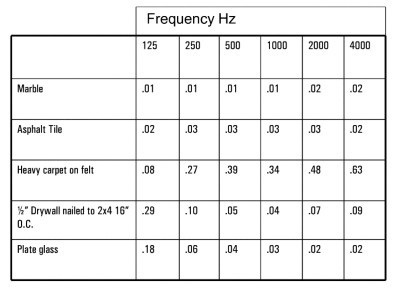## Typical Application

Consider a conference room that was built with carpet flooring and drywall ceilings and walls. The speech intelligibility in the room was very poor and something needed to be done to correct the problem. The room was constructed primarily of drywall with carpet flooring. Using the equations for reverberation time and absorption as well as a more complete table of absorption coefficients we could estimate the reverberation time of the space. However, to get a more accurate baseline figure of the reverberation time we need to take some measurements in the space.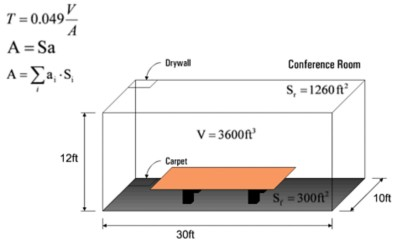## Reverberation Testing

In this case we chose the LabVIEW Sound and Vibration Toolkit and the NI 4472 to conduct an impulse test using a high voltage electrode that generates a spark upon a digital trigger. Other techniques include firing a gun, bursting a balloon or simply a loud clap of the hands. The source was positioned at the front of the room in which the speaker usually resides. Microphones were then used throughout the space to gather the response of the disturbance upon a trigger received from the source. The signal was then digitized with the 4472 and then sent to a PC for processing. We can then calculate the time for the sound pressure to decay by 60 dB. Impulse testing can give a great deal of information regarding the quality of a space. In this case we used the data to measure the reverberation time of the space however an impulse test can also identify problematic reflections or a lack of reflections that must be compensated for.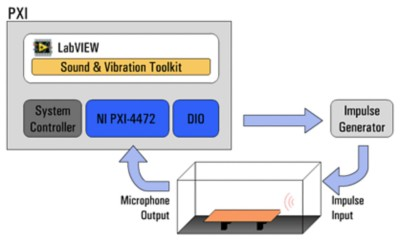## Data Processing

Once the data is gathered we define the point at which the sound pressure falls by 60 dB from the original impulse. To retrieve the digitized data from the 4472 we used the DAQmx Read function. After this data was available for processing we scaled the voltage to pascals (Pa) using the SVL Scale Voltage to EU function and measured the time taken for the sound level to fall by 60 dB using the native LabVIEW functions as well as the SVT Leq Sound Level VI.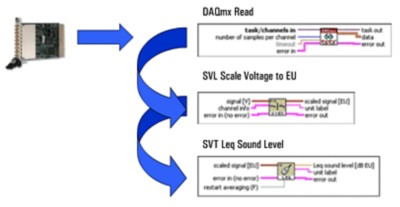## Design Correction

Using the impulse method of testing we found the room to have a reverberation time of about 1.15 seconds. As we suspected, this time is too long for the intended purpose of the room. We can now attempt to design for a space with a much more pleasing reverberation time. Using the general formula for reverberation time T = 0.049V/A and that the volume of the room is 3600 ft^3 we can see that the room has an equivalent absorption area of 153 ft^2. To achieve a more desirable reverberation time of about 0.6 seconds we’ve found we need to increase the equivalent absorption of the room to approximately 294 units.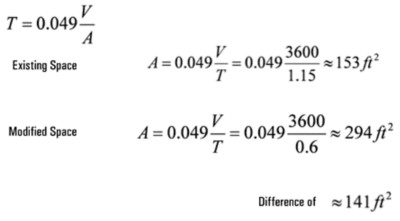## Design Considerations

Some rooms rely on ceiling reflections to project sound to the far reaches of the space therefore the ceiling is usually avoided when considering the placement for acoustic material. However in our conference room the listener will rely mostly on direct sound from the source and will not need ceiling reflections to clearly hear the source. Remember also that the conference table serves as another surface reflecting sound to the listeners throughout the room. Therefore we have chosen to place material on the ceiling keeping the walls free of clutter. In order to increase the absorption area we must select a material with a larger absorption coefficient than the drywall that currently exists. Therefore a common material such as ceiling tile was chosen that has an absorption coefficient of 0.68 @ 1000 Hz. Listed above are a few of the other considerations that must be taken into account when compensating a room for poor reverberation response.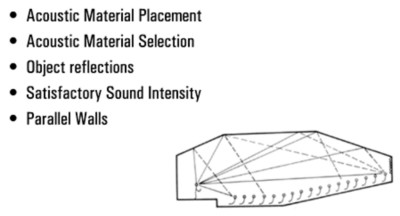## Verification and Summary

300 ft^2 of perforated cane fiber were added bringing the total theoretical equivalent absorption area of the room to be 345 ft^2. This number being beyond the 294 mark that was calculated earlier should ensure that speech intelligibility is retained. Now that we have modified the space to obtain a shorter reverberation time another measurement must be taken. Adding 300 ft^2 of perforated cane fiber 1” on metal frame brought the reverberation time to just at T = 0.61 seconds. This time is ideal for the purpose of our room. Remember that acoustic design is not a complete science. We had expected the reverberation time to be shorter than this given the fact that we added more absorption material than we had calculated for. Another factor to remember is that by adding the dropped ceiling, we also changed the geometry of the room and this did impact how the room responds to the same impulse.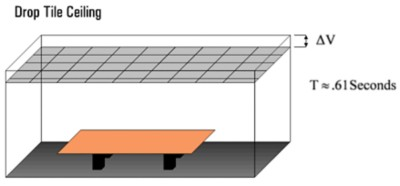Reverberation Time is the amount of time required for the sound pressure in a space to decay 60 dB, or to one millionth of the original energy Absorption coefficients are used to help predict or design for a space with a desired reverberation response. Reverberation Time is measured by collecting data throughout a space of the response to an impulse. Reverberation time testing is a measurement made simple through the use of National Instruments hardware and software. The impulse response of a space can be acquired using tools such as the 4472 and the reverberation time can be calculated in software using LabVIEW and the Sound and Vibration Toolkit.